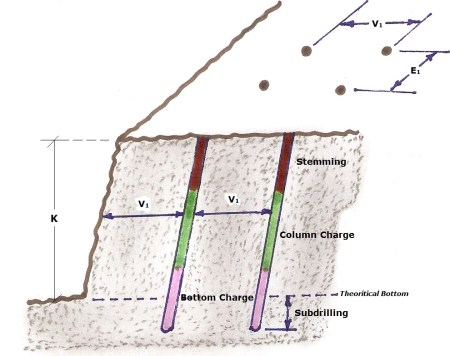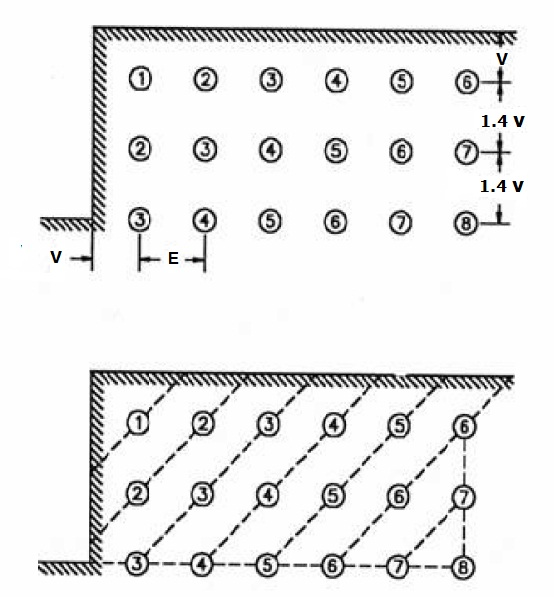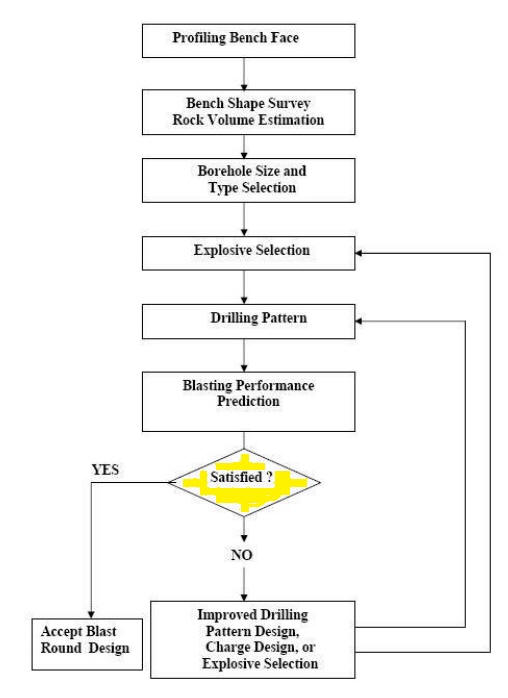Bench Blasting

Bench Blasting Basics

Bench blasting consists of drilling holes in the rock at depths, in diameters, and at spacing so that the ANFO (which is a mixture of Ammonium Nitrate[fertilizer] and Fuel Oil [diesel fuel]) as a column charge,together with a bottom charge, can fracture the rock in a controlled manner.

Note : Bottom charge is used as a primer and booster charge to the column charge ANFO (Primer charge is used to intiate other explosives, and contains a detonator or a detonating cord. A booster does not contain a detonator, its purpose is to maintain or intensify the explosive reaction along the blast hole. A bottom charge is mostly a dynamite – Dynamite is a mixture of highly sensitive nitroglycerin with sawdust, powdered silica, or most commonly diatomaceous earth, which act as stabilizers).

Rock is a material which has widely varying charachteristics, and can vary in strength properties, specially when containing faults,cracks, or intercalated by other rock types, which affect its resistance to the effect of explosives.

It is found that the available theoretical basis for charge calculations is empirical. Empirical values have been arrived at by test-blasting and the accumulation of the experience. The unit which would appear to correspond most simply to the blasting charachteristics in rock (of a certain explosive) is a certain amount of explosive of a certain strength in relation to the volume of rock . That is the specific charge (blasting ratio) in kg/m3, which is taken 0.4 kg/m3 as a standard value for purpose of test-blasting.

The specific charge is an excellent unit for the calculation of charges. But the order and distribution of the drill holes along the surface of the round, and the distribution of the total charge in each drill hole, are also extremely important. The initiation method and firing plan, are also of the greatest importance.

Much is said about blasting in theory and blasting in practice. The capacity of the skilled professional to remember experience which he then applies in his daily work is, in my opinion, a very advanced and qualified form of theory. The values based on experience – empirical values – which have been accumulated through the years, and provide the basis for the development of relationships and formulas for charge calculations, realy make up the same thing.

Nomenclature: (Figure 1)

¨     Round : : is one stage of blasting , producing an amount of fragmented rock (m3).

¨     Burden (V) : shortest distance (m) between the bottom of the drill hole and the free face, against which the breakage is expected.

¨     Vmax = maximum burden in meters.

¨     V1  = Practical burden in meters.( V1 should realy be calculated at right-angles to the drill hole but, for practical reasons, the horizontal distance is used.)

¨     E1   = practical hole spacing in meters.

¨     d   = bore hole diameter in meter.

¨     K   = bench hight im meters.

¨     U   = subdrilling in meters.

¨     Qb  = bottom charge in kg.

¨     Qp  = column charge in kg.

¨     Q (total charge) = Qb + Qp .

¨     q   = specific charge in kg/m3 ( explosives consumption per on cubic meter of rock).

¨     Specific Drilling (b) : drilled meters per on cubic meter of excavated rock (drilled meters/m3).

Rock Conctant (C) or Blasting Ratio or Blastability : is a charge needed by weight for breaking one cubic meter of rock kg/m3 (breakability of rocks, its extreme values : 0.2 for easy blasted, 1 for difficult, and 0.4 kg/m3 as a standard value, which is considered directly for blast-test

Note : Hino proposed that blastability (named as Blasting Coefficient(BC) by him) is the ratio of compressive strength (CS) to tensile strength (TS) of rockmass, which may be given as follows.

BC = CS / TSFigure 1 : Bench Blasting Geometry

Charge calculation

·         Maximum theoretical burden Vmax = 45 x d … where 45 is a factor k taken for test blasting purposes.

·         Subdrilling U = 0.3 x Vmax

·         Hole depth H = K + U + 0.05 (K + U)

·         Note : 0.05 (K + U)  for 5 cm/meter drill hole for hole inclination of 3:1

·         F = 0.05 + 0.03 H … where is defined as : (faulty drilling – heave factor).

·         Note : 0.05 is a 5 cm application error, and 0.03 is 3 cm/m drill hole.

·         Practical burden V1 = Vmax – F

·         Practical hole spacing E1 = 1.25 x V1

·         Concentration of bottom charge Qbk = d2/1000

·         Height of bottom charge hb = 1.3 x Vmax

·         Weight of bottom charge Qb = hb x Qbk

·          Concentration of column charge Qpk = 0.4 – 0.5 Qbk

·         Height of column charge hp = H – (hb + ho)  .. where  ho is uncharged section.

·         Uncharged Section ho = V1  .. equals Vmax in certain cases.

The following example shows how calculations is carried out

Conditions given :

Bench Height  K          = 12 m

Width of round B        = 20 m

Drill hole diameter d    = 64 mm

Solution :

1.    Vmax = 45 x d = 45 x 64 =2880 cm = 2.88 m

2.    U     = 0.3 x Vmax = 0.3 x 2.88 = 0.864 (0.9 m)

3.    H     = K + U + 0.05 (K + U)    = 13.54 (13.6 m)

4.    F     = 0.05 + 0.03 x H = 0.05 + 0.03 x 13.6 = 0.46

5.    V1   = Vmax – F = 2.42 (2.4 m)

6.    E1   = 1.25 x V1 = 3 m

7.    Number of hole spacings = B/E1 = 6.6 (7)

8.    E1 corrected = B/7 = 20/7 = 2.86 m

9.    Qbk = d2/1000 = 642/1000 = 4100/1000 = 4.1 kg/m

10.  hb = 1.3 x Vmax = 3.7 m

11.  Qb  = hb x Qbk = 15.2 kg

12.  Qpk = 0.4-0.5 Qbk = 0.5 x 4.1= 2.05 .. approx. 2 kg/m

13.  ho  = V1 = 2.4 m

14.  hp  = H – (hb + ho) = 13.6 – (3.7 + 2.4) = 7.5 m

15.  Qp  = hp x Qpk = 15 kg

16.  Qtotal = Qb + Qp = 15.2 + 15 = 30.2 kg

17.  q  = [( holes/row) x Qtotal] / V1 x K x B = (8 x 30.2)/(2.4 x 12 x 20) = 0.42 kg/m3

18.  b = [(holes/row) x H] / (V1 x K x B) = 0.19 drilled meters/m3

Summry of important data

 Specific drilling b Specific charge kg/m3 Column Charge Bottom Charge kg Hole spacing m Burdenm Hole depth m Bench Height m Kg/m Kg 0.19 0.42 2.0 15.0 15.20 2.86 2.40 13.6 12.0

The test blasting of one ore more rows is a good way to obtain some impression concerning the blasting charahteristics of the rock. Where it is desirable, at least in connection with production blasting, to utilize the drill holes as much as possible, then the calculations can be adjusted most easily by changing the factor k in the relationship :

Vmax = k x d ...... (see figure 2)

A change of this factor from k = 45 x d to other values changes the specific charge in the bottom section, but also modifies the round as a whole.

Charge calculations should otherwise be carried out in the same way. This means that the calculation system can be adapted to all types of rock, no matter what their blasting charachteristics may be.Figure 2

Hole spacing and timing

The top view of the rock blasting geometry is shown in Figure 3. Notice the distance V is still the distance to the free face. The distance, E, or spacing of the holes is a function of the burden V .

The spacing of the holes and the timing (or delay) of the holes are part of the blasting design. The bottom illustration in Figure 3 shows how the blast is delayed by the sequencing numbers. Each hole may be blasted milliseconds apart to control the blast. The row-to-row shots are certainly time delayed.

An initiation system transfers the detonation signal from hole to hole at precise times. Plastic shock tubes or electric caps using a timing system are generally employed. A shock tube is non-electric, instantaneous, and has a thin reactive powder that propagates the shock wave signal.

The timing or delay minimizes the pounds of explosive per delay period. This can significantly control noise and vibration effects. It would be a disaster if all the holes went off at the same time.

The design variables of burden, stemming, subdrill length, spacing, and timing are selected to maximize fragmentation and to minimize excessive vibration, air blast, and flyrock.Figure 3

____________________

References :

·         R.McAdam & R.Westwater/ Mining Explosives- London 1958

·         Rune Gustafsson/ Swedish Blasting Technique- Gothenburg, Sweden  1973

·         Rock Blasting Document 208 : http://www.dot.state.oh.us/Pages/Home.aspx

14-Apr-11

Atef HelalFigure 4

Procedure of blast round design and evaluation of blasting Performance

(Reproduced from : http://miningandblasting.wordpress.com/)

14-Apr-11

Atef Helal

__________________

Index